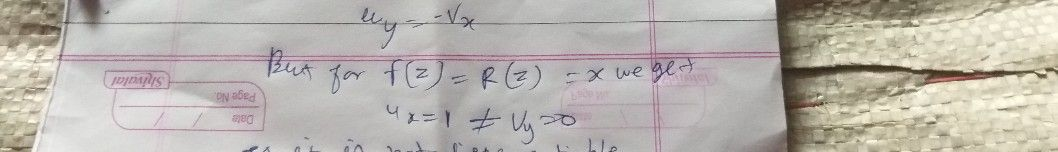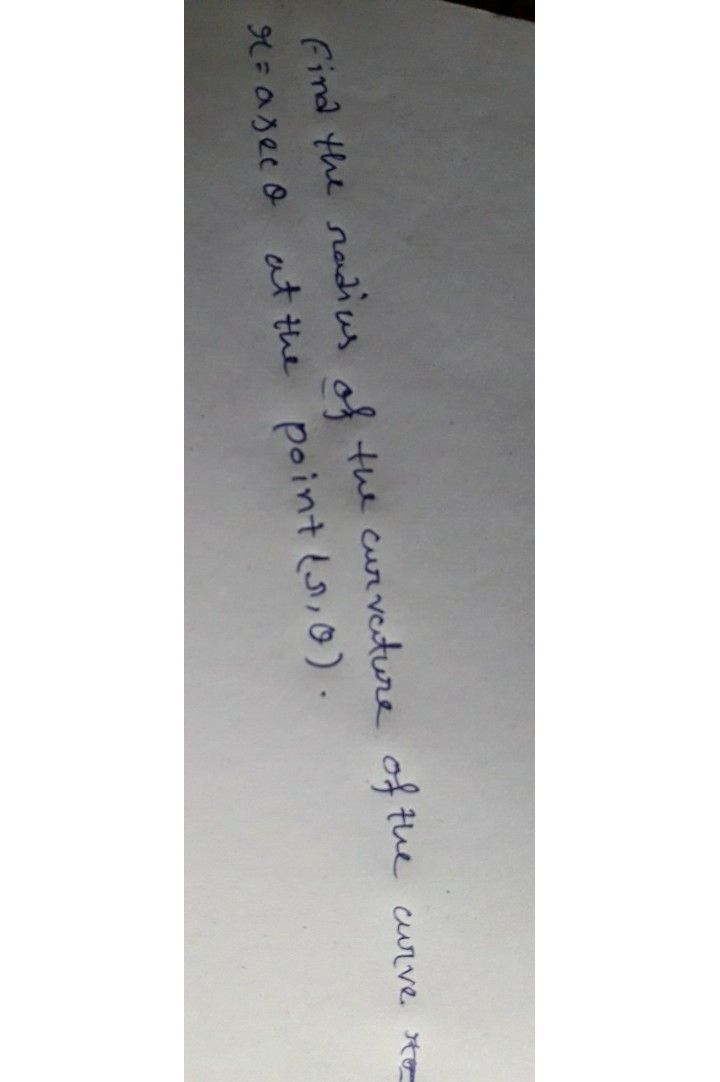Symbol
Problemfind the sradius of the curveture of the curve $x\left(=a$ $Q$ at the point $in+\left(3,θ\right)$
Calculus
SolutionQanda teacher - Arushiplz send complete and clearl picStudent